Back to Nautical Displacement Buoyancy FWA Movement of Centre of Gravity Angle of Loll Curves of Stat. Stability List Free Surface Effetcs Trim

Stability

Trim

Trim

For a rectangular box shaped vessel, when a weight is added on to one side the vessel would list to that side.

If however the weight is added either behind or ahead of the of the midship area but within the centre line partition of the ship then the vessel would get tilted either forward or aft.

This tilting is known as TRIM

Thus trim is the longitudinal equivalent of list.

However there is a fundamental difference in the way the List and Trim are noted. List is as we know expressed in degrees, trim may be measured also in degrees but the expression is in Feet or Metres.

Thus Trim may be defined as the difference between the draft at the fore perpendicular and the draft at the aft perpendicular.

Unlike list which is stated as Port or Starboard, Trim is stated as Positive or Negative – more usually as Trim – meaning trimmed by stern, taken as positive. And Trim by head – meaning negative and that the draft ahead is more than the draft astern.

Moment to Change Trim 1 cm (MCTC)

Now we have seen that to change the Trim we need to move weights in the fore and aft line of the ship.

This then brings about a moment, and the moment required to change the trim by 1 cm is given by:

MCTC = (W x GMl) / 100 x L

Where W is the displacement of the vessel in tonnes

GMl is the longitudinal metacentric height (m)

L is the Length between perpendiculars (m)

Centre of Floatation

This is the imaginary point where the ship pivots. It is the centre of gravity of the water plane area. The centre of Floatation is also referred to as the ‘Tipping Centre’

A box shaped vessel with a rectangular water plane area would have its centre of floatation amidships, whereas on a ship shaped vessel the centre of floatation would be either slightly forward or abaft of amidships.

Remember all trimming moments are taken about the centre of floatation, since it is around this point that the vessel pivots.

Change of Trim

This is the difference between a earlier trim and the latest trim. For example the trim that the vessel had on departure and the proposed trim that the vessel would have on arrival at the destination port.

Longitudinal Metacentre (ML)

In the manner of the Metacentre, the Longitudinal Metacentre is the point of intersection between the verticals passing through the centre of buoyancy when the vessel is on an even keel and when the vessel is trimmed.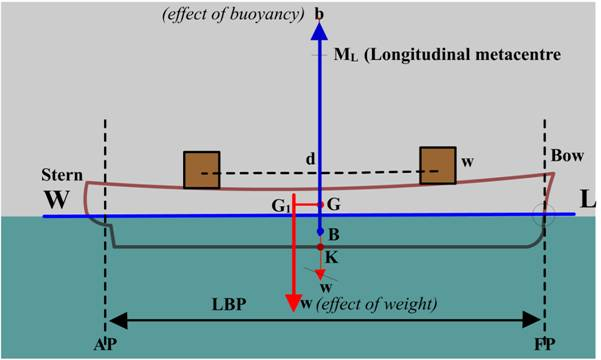Longitudinal Metacentric Height (GML)

This is the vertical distance between the Centre of gravity of the vessel and the longitudinal Metacentre

In the above figure we see that

GG1 = (w x d) / W

Or W x GG1 = w x d

Trimming moment = W x GG1 = w x d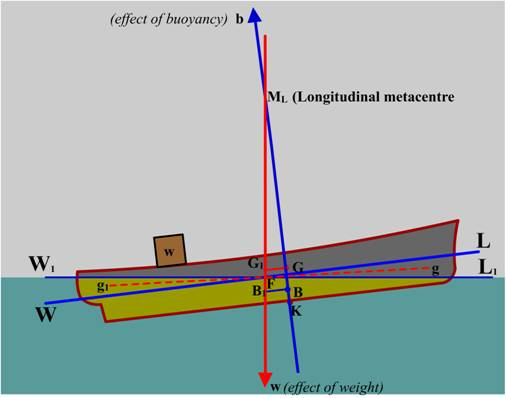The vessel trims until G and B come in the same vertical line again

Also take note that since the distance BG is very small as compared to BML, sometimes BML may be substituted for GML in calculations, without any appreciable error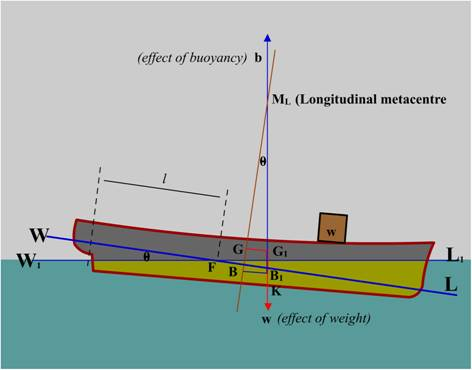Tan θ = trim / LBP = t /L where, trim in cm and LBP is in metres

Tan θ = GG1/ GML = (w x d) (W x GML) because

GG1 = (w x d)/ W

T/ 100L = (w x d) / (W x GML)

T = (w x d) x 100L (W x GML)

T = (w x d) / MCTC = Trimming Moment / MCTC

Where Trim obtained will be in cm.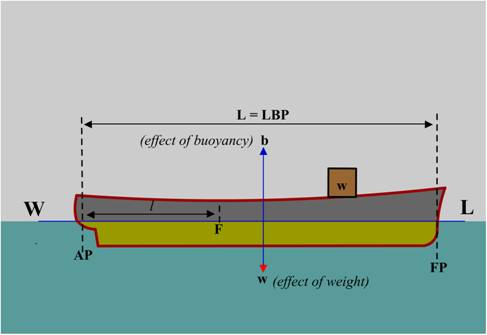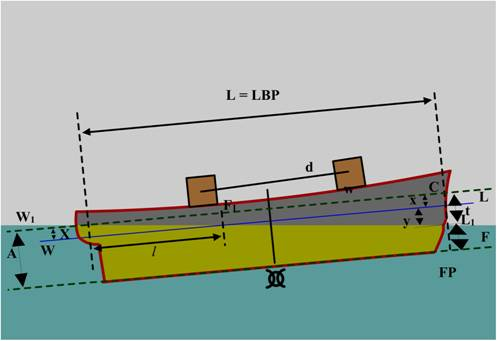Trim = t / 100L

Where L = LBP

T – trim in cms

To find the change of draft forward and aft due to change of trim

Change of trim = Trimming Moment / MCTC

Change of draft aft (cm) = (l x change of trim) / L

Where:

L is the distance of the centre of floatation from the aft perpendicular (m)

L is the LBP (m)

Change of draft forward (cm) = change of trim – change of draft aft

Or

Change of draft aft (m) = (L-l) / L x Change of Trim

Loading / discharging at the centre of floatation will produce no change of trim but the draft will only change

Only if the weight is shifted to either forward d or aft will we get a trimming effect.

Shifting a weight will on the other hand give only a change of trim but not of draft

Similarly discharging can be considered as shifting to centre of floatation first and then taking the load off the ship

So the two components to be calculated are:

a. Change of draft

b. Change of trim

Then we go on to calculate the draft forward and aft

Hence  calculate these problems as follows:

Bodily sinkage = W / TPC

Then calculate the change of trim

Change of trim (cm) = Trimming Moment / MCTC

3. Then calculate the change of aft draft – change of aft draft (cm) = l / L x COT

4. Then calculate the change of draft forward – change of draft forward (cm) = COT – change of draft aft

OR

(L-l) / L x COT